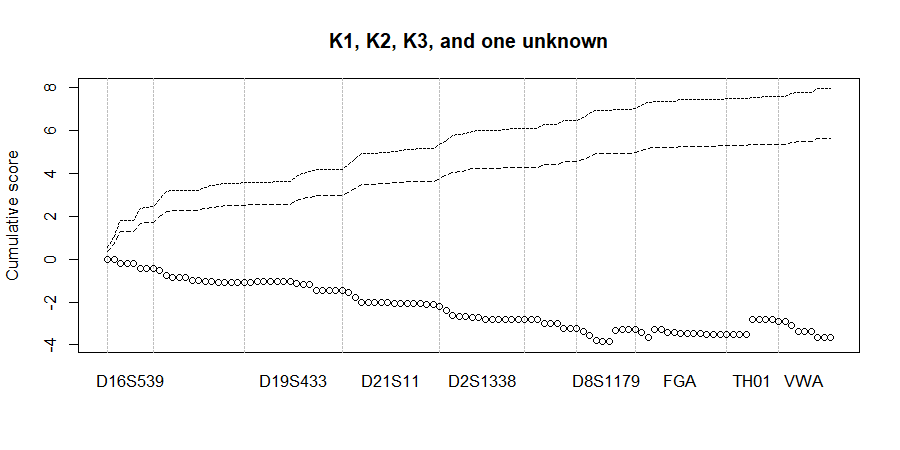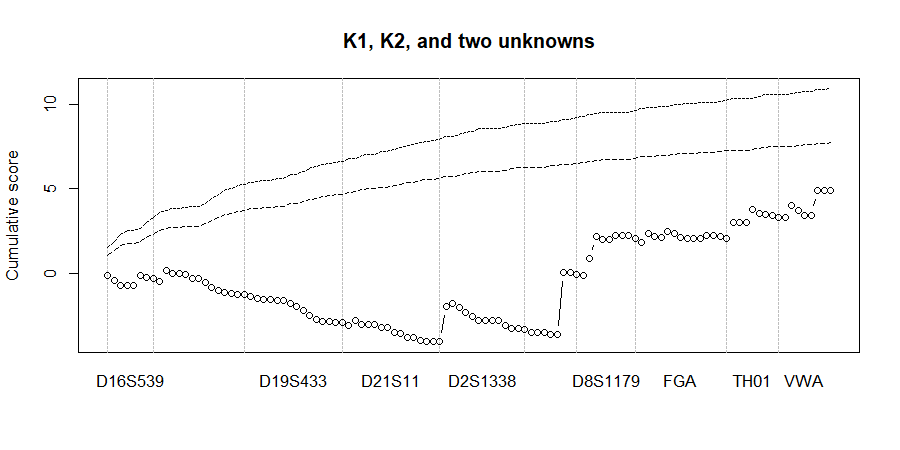# DNAmixtures

## Statistical analysis of DNA mixtures with artefacts

by Therese Graversen

# Tutorial

Below we demonstrate a few features of DNAmixtures. For a more thorough walk-through, see for instance the example analysis in Case analysis using the DNAmixtures package or the detailed discussions in Graversen (2014).

The manual pages contains detailed descriptions of all functions available and a brief presentation of the statistical model. Further details on the model and its parameters can be found in Cowell et. al. (2015).

## Setting up a DNA mixture model

```data(MC15)
MC15[MC15\$marker == "TH01",]
```
```##    marker allele height K1 K2 K3
## 35   TH01    7.0    727  1  0  0
## 36   TH01    8.0    625  1  0  0
## 37   TH01    9.0      0  0  2  0
## 38   TH01    9.3    165  0  0  2
```

The allele frequencies are also specified in a `data.frame`, this one containing variables `marker`, `allele`, and `frequency`. The range of alleles in this dataset determines the range of alleles used in the model.

The US-Caucasian allele frequencies are included in the package:

```data(USCaucasian)
USCaucasian[USCaucasian\$marker == "TH01",]
```
```##    marker allele   frequency
## 22   TH01    5.0 0.001659967
## 23   TH01    6.0 0.231785364
## 24   TH01    7.0 0.190396192
## 25   TH01    8.0 0.084438311
## 26   TH01    9.0 0.114237715
## 27   TH01    9.3 0.367542649
## 28   TH01   10.0 0.008279834
## 29   TH01   11.0 0.001659967
```

As an example, let us create a model for the single DNA mixture MC15. The prosecution hypothesis could be that K1, K2, and K3 have contributed to the trace together with an unknown contributor U1, that is k = 4 contributors in total. We set the detection threshold C to 50 rfu.

```data(SGMplusDyes)
mixHp <- DNAmixture(list(MC15), k = 4, K = c("K1", "K2", "K3"), C = list(50),
database = USCaucasian, dyes = list(SGMplusDyes))
```

We can plot the peak heights by

```     plot(mixHp, epg = TRUE, dyecol = list(c("blue", "green", "black")))
```## Estimating model parameters

Parameters can, for instance, be estimated by maximum likelihood. Here we using the parameter `p` as the initial value for optimisation.

```     p <- mixpar(rho = list(30), eta = list(34), xi = list(0.08),
phi = list(c(K1 = 0.71, K3 = 0.09, K2 = 0.19, U1=0.01)))
mlHp <- mixML(mixHp, pars = p)
mlHp\$mle
```
```##       rho     eta       xi     phi.U1   phi.K1    phi.K2   phi.K3
## 1   34.24   26.67   0.0737   0.008459   0.8205   0.04734   0.1237
```

Relying on asymptotic normality, we can compute standard errors for the estimates as follows.

```var15Hp <- varEst(mixHp, mlHp\$mle,
npars = list(rho = 1, eta = 1, xi = 1, phi = 1))
summary(var15Hp)
```
```##             Estimate    StdErr
## rho.1      34.237686   7.13108
## eta.1      26.668447   5.61843
## xi.1        0.073699   0.01441
## phi.U1.1    0.008459   0.01853
## phi.K1.1    0.820501   0.02015
## phi.K2.1    0.047343   0.01361
## phi.K3.1    0.123698   0.01532
```

## The likelihood function and likelihood ratios

To compute a likelihood ratio, we firstly need to formulate an alternative hypothesis. In this case, we substitute K3 for an unknown contributor.

```mixHd <- DNAmixture(list(MC15), k = 4, K = c("K1", "K2"),
C = list(50),
database = USCaucasian)
p <- mixpar(rho = list(30), eta = list(34), xi = list(0.08),
phi = list(c(K1 = 0.71, U1 = 0.09, K2 = 0.19, U2=0.01)))
mlHd <- mixML(mixHd, pars = p)
mlHd\$mle
```
```##       rho    eta        xi    phi.U1    phi.U2   phi.K1   phi.K2
## 1   25.54   35.8   0.07192   0.08115   0.08114   0.7983   0.0394
```

There is no designated function for computing a likelihood ratio. However, we can easily compute the weight-of-evidence against K3, which is simply log10 of the likelihood ratio.

The (natural) log likelihood under each of the hypotheses is
```mlHp\$lik ## K1 & K2 & K3 & U1
```
```##  -271.8021
```
```mlHd\$lik ## K1 & K2 & U1 & U2
```
```##  -297.7915
```

Taking their difference, we get the log likelihood ratio. This is then divided by log(10) to get the logLR on log10 scale instead:

```(mlHp\$lik - mlHd\$lik)/log(10)
```
```##  11.28704
```

## Mixture deconvolution

If a proposed hypothesis includes unknown contributors, it may be relevant to investigate likely allocations of genotypes for these individuals.

Consider thus marker TH01 and the individual U1. We find the set of all genotypes with a posterior probability above ```pmin = 0.008``` given the observed peak heights.

```setPeakInfo(mixHp, mlHp\$mle)              ## condition on observed peak heights
mp <- map.genotypes(mixHp, pmin = 0.008,  ## find the best set of genotypes
markers = "TH01")
summary(mp)
```
```##
## TH01:
##      U1.1   U1.2   Prob
## 1    9.3     NA    0.247461
## 2    7      9.3    0.154065
## 3    9.3    9.3    0.138326
## 4    7       NA    0.136296
## 5     NA     NA    0.108608
## 6    8      9.3    0.067610
## 7    8       NA    0.059862
## 8    7      7      0.042352
## 9    7      8      0.037264
## 10   8      8      0.008155
##
## Total probability: 1
```

The `NA` indicates a dropped out allele; the individual has a high probability of exhibiting dropout, which is not surprising given that the corresponding estimated mixture proportion was very low. Further inspection shows that all other predicted alleles are, in fact, masked by known contributors.

## Challenging the interpretation of a mixture

It is important to justify that any model under consideration can also suitably explain the data. The statistical framework of `DNAmixtures` enables the addressing of such issues.

### Simulating peak heights

The perhaps simplest way of comparing the observed peak heights to their distribution under a particular model is to compare to a set of simulated peak heights.

```     ## Compare observed peak heights to peak heights simulated under the model
sims <- rPeakHeight(mixHp, mlHp\$mle, nsim = 100, dist = "conditional")
oldpar <- par(mfrow = c(2,5), mar = c(2,2,2,0))
boxplot(mixHp, sims)
``````     par(oldpar)
```

In fact, we could also produce a plot using the quantiles in the exact distribution rather than relying on simulations; see for instance Graversen (2014) and Graversen and Lauritzen (2014)

### Quantile-quantile plots

The quantile-quantile plot is a convenient way of assessing whether the observed peak heights follow their assumed distribution. If the distribution is adequate, the points should follow the diagonal.

```qqpeak(mixHp, pars = mlHp\$mle, dist = "conditional")
```### Prequential monitor plots

A prequential monitor may be used for assessing the ability of the model to correctly predict the presence or absence of a peak for a particular allele. For each position in the EPG in turn, we compare whether the model predicts a peak or not to whether a peak has been observed.

We look out for upwards jumps, as they occur when the model has predicted the opposite of what was observed. The monitor (circles) crossing the upper 95% or 99% bounds indicates that mis-predictions occur more frequently than expected.

```     pr <- prequential.score(mixHp, pars = mlHp\$mle)
plot(pr, main = "K1, K2, K3, and one unknown")
``````     pr <- prequential.score(mixHd, pars = mlHd\$mle)
plot(pr, main = "K1, K2, and two unknowns")
```In both plots, the prequential monitors stay well within the upper bound, indicating that the presence and absense of peaks in the EPG is adequately explained by either of the hypotheses under consideration.

DNAmixtures provides easy access to a wide range of statistically interesting quantities.

As an example, we evaluate and plot the profile likelihood for the proportion of DNA contributed by individual K3 under the hypothesis that the donors are K1, K2, K3, and an unknown contributor.

```proflik <- function(x){
mixML(mixHp, mlHp\$mle,
constraints = function(p)p[[1, "phi"]][["K3"]],
val = x)\$lik
}
proflik <- Vectorize(proflik)

curve(proflik(x)-mlHp\$lik, 0.01, 0.2, n=19,
xlab = expression(phi[K3]), ylab = "Profile likelihood")
```The profile likelihood illustrates clearly that the data support a non-zero contribution from K3.